# Second order analysis - Questions Part 2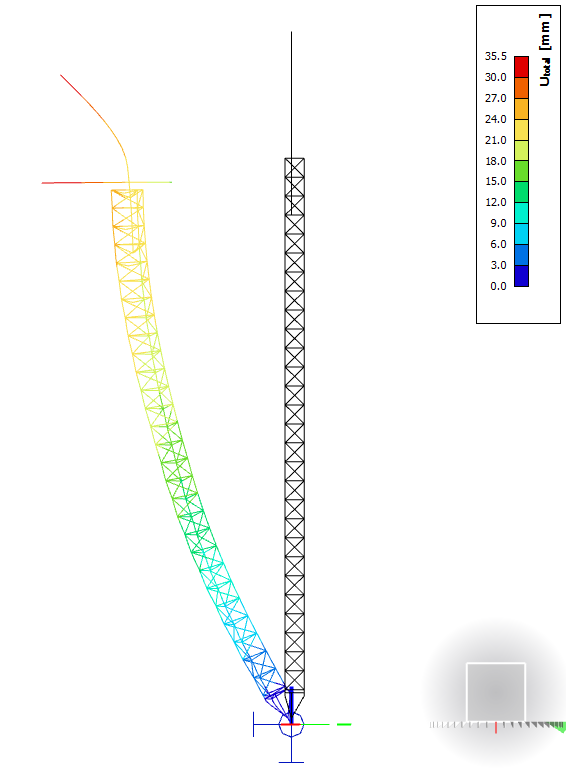Second order analysis takes into account how the structure deforms while loads are being applied on it. Numerically speaking, all load is divided into smaller portions and for each load portion, the stiffness of the structure changes.

Second order effects are also called P-Δ and p-δ effects, and in SCIA Engineer (among other places) these effects are also referred to as Geometrical nonlinearity.

Here are some common questions from users that our Support team often needs to address.

## I have performed a second order analysis including imperfections, why is SCIA Engineer still doing buckling verifications?

Verifications by second order analysis can only replace flexural buckling checks according to the code (i.e. sideways loss of stability in y- or z- direction). Any torsional stability failure modes still need to be verified according to the methods given in the code.

This is because of the element formulation of 1D members (beams and columns) in the FEM solver. 1D elements cannot distort on the level of cross-section, their section remains planar and perpendicular to the member system line. Therefore, warping deformation is not captured by the model itself, hence, lateral torsional and torsional failure modes cannot be predicted (regardless of the number of FEM elements along each beam). Such failure modes need to be verified by design calculations.

## What does the functionality ‘geometrical nonlinearity’ mean?

Geometrical nonlinearity refers to a second-order calculation. When you execute an analysis while this setting is turned on, the structure will be deforming as load is being applied and the load steps and numerical method for nonlinear calculation will be taken as defined in the Solver Settings. Therefore, results will be different to a linear analysis, regardless if initial imperfections are applied or not. However, to properly capture important nonlinear effects, it is advisable that imperfections be used.

NOTE: Geometrical nonlinearity is taken into account in nonlinear analysis, which is in turn executed for nonlinear combinations. Second order effects are not present in the linear analysis results: linearly solved load cases are superposed to obtain the results of linear combinations. In nonlinear context, the principle of superposition does not apply, because the effects of loads influence the effects of other loads.

## How should I calculate the imperfection amplitude when using a buckling shape as an imperfection?

### FramesEN 1993-1-1 gives guidance in §5.3.2 (11) on how to calculate the amplitude of a unique imperfection shape derived directly from stability analysis (ηinit). This method is valid for frames, and requires that the user derive the amplitude manually and input it in SCIA Engineer. The choice of an imperfection shape is also up to the user.

This method of managing imperfections requires that the user define a load combination for the stability analysis itself and visually review the obtained instability checks. The user should select the lowest critical buckling shape that represents the overall deformation of the structure in the direction being investigated in the nonlinear load scenario that will follow. The instability shape and its amplitude are again inputted per nonlinear combination.

For the derivation of the imperfection amplitude, the user would have to obtain a few inputs from the FE model, from linear and stability analysis results, as well as from the Steel Code Check.

The imperfection is derived as follows: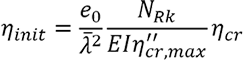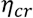is the shape of the elastic critical buckling mode, hence, the term before it is the amplitude valuefor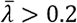is the relative slenderness of the whole structure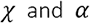reduction and imperfection factor, as derived for the critical section; these can be taken from the Steel Code Check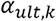is a factor which, if applied on the loads in a linear scenario, would make the most compressed member reach its characteristic section resistance NRkthe elastic critical buckling factor for the stability combination and selected buckling shape. For consistency, the stability combination should contain the same loads as analysed in the nonlinear combination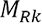the characteristic moment resistance of the critical cross-section, calculated for the relevant section class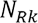the characteristic axial force resistance of the critical cross-section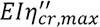(whole term) is the bending moment due to ηcrat the critical cross-section.

When we interpret the above, we see that we need to determine:

• the critical cross-section in the structure (where the load effects lead to a maximum utilisation ratio in linear context),
• the most compressed member in the structure.

αult,k, MRk, NRk, χ  and α can be obtained from the Steel Code Check. αcr and (EIη"cr,max) can be obtained from the stability analysis results.

### PlatesFor structures modelled by 2D plate elements, a similar procedure applies, but the amplitude of imperfections should be determined from  §C5 of EN 1993-1-5. Again, to properly capture all potential buckling failure modes, one should execute nonlinear analysis with second-order effects and initial imperfections.

Due to the versatility of plated element models, in theory, all failure modes can be captured with appropriate:

• meshing,
• element formulation for bending and shear,
• and inclusion of imperfections.

This is different from frame structures, where additional verifications are required for lateral-torsional and torsional buckling.
§C5 of EN 1993-1-5 essentially two possibilities for the definition of imperfections:

a. An explicit modelling of both geometric and structural imperfections:

• geometric imperfections can be approximated as buckling shapes, with amplitudes taken as 80 % of the fabrication tolerances;
•     structural imperfections (e.g., residual stresses) may be represented by a stress pattern expected from the fabrication process with amplitudes equal to the expected (mean) values.

b. Modelling the imperfection shape and amplitude according to a simplified approach given in table C.2. In this case, both geometric and structural imperfections are represented by equivalent geometric imperfections.

Table C.2 gives amplitude values per type of component that are simple to derive. However, the chapter specifies that different types of geometrical imperfections may need to be combined together: in addition to the main imperfection, other imperfection shapes should be represented (in addition) with 70 % of their recommended amplitude value. SCIA Engineer only allows a single buckling shape to be used as imperfection: if needed, accompanying geometric imperfections can be substituted by appropriate fictitious forces acting on the structure.

If you missed the 1st part of the article, you can find it here.